Courses

# Test: Time And Distance - 1 (2019-2016)

## 10 Questions MCQ Test UPSC Topic Wise Previous Year Questions | Test: Time And Distance - 1 (2019-2016)

Description
This mock test of Test: Time And Distance - 1 (2019-2016) for UPSC helps you for every UPSC entrance exam. This contains 10 Multiple Choice Questions for UPSC Test: Time And Distance - 1 (2019-2016) (mcq) to study with solutions a complete question bank. The solved questions answers in this Test: Time And Distance - 1 (2019-2016) quiz give you a good mix of easy questions and tough questions. UPSC students definitely take this Test: Time And Distance - 1 (2019-2016) exercise for a better result in the exam. You can find other Test: Time And Distance - 1 (2019-2016) extra questions, long questions & short questions for UPSC on EduRev as well by searching above.
QUESTION: 1

### X, Y and Z are three contestants in a race of 1000 m. Assume that all run with different uniform speeds. X gives Y a start of 40 m and X gives Z a start of 64 m. If Y and Z were to compete in a race of 1000 m, how many metres start will Y give to Z? 

Solution:

x gives y a start of 40 m.
x gives z a start of 64 m.
Let they all complete the race in t hours.
So, x's speed = (1000/t)
y's speed = (960/t)
z's speed = (936/t)
Again, Let y gives z a start of x m.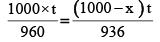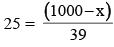x = 1000 – 975 = 25 m.

QUESTION: 2

### When a runner was crossing the 12 km mark, she was informed that she had completed only 80% of the race. How many kilometres was the runner supposed to run in this event? 

Solution:

Let the runner suppose to run n km.
then,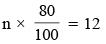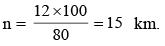QUESTION: 3

### Two persons, A and B are running on a circular track. At the start, B is ahead of A and their positions make an angle of 30° at the centre of the circle. When A reaches the point diametrically opposite to his starting point, he meets B. What is the ratio of speeds of A and B, if they are running with uniform speeds? 

Solution:

B is 30° head to A
Total angle covered by A = 180°
Total angle covered by B = 180° – 30° = 150°
Required ratio = 180° : 150° = 6 : 5

QUESTION: 4

A train 200 metres long is moving at the rate of 40 kmph. In how many seconds will it cross a man standing near the railway line?



Solution:

Length of train = distance = 200 m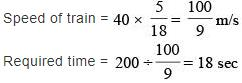QUESTION: 5

The figure drawn below gives the velocity graphs of two vehicles A and B. The stright line OKP represents the velocity of vehicle A at any instant, whereas the horizontal straight line. CKD represents the velocity of vehicle B at any instant. In the figure, D is the point where perpendicular from P meets the horizontal line CKD such that PD = 1/2 LD: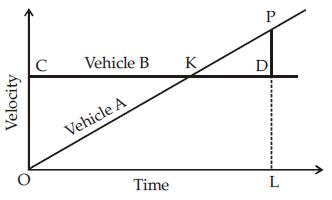What is the ratio between the distances covered by vehicles A and B in the time interval OL?



Solution: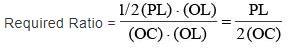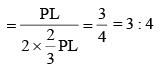QUESTION: 6

Average hourly earnings per year (E) of the workers in a firm are represented in figures A and B as follows: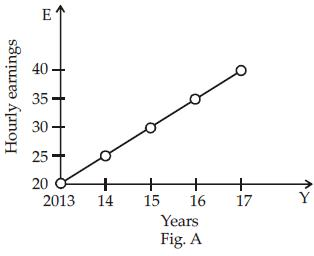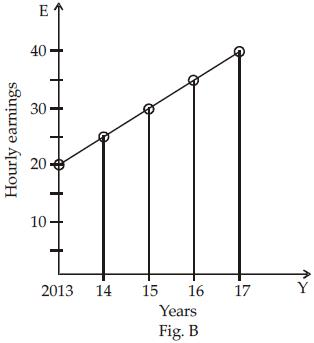From the figures, it is observed that the



Solution:

We can see from graph slopes of both graphs are same

QUESTION: 7

A freight train left Delhi for Mumbai at an average speed of 40 km/hr. Two hours later, an express train left Delhi for Mumbai, following the freight train on a parallel track at an average speed of 60 km/hr. How far from Delhi would the express train meet the freight train?



Solution:

Distance travelled by freight train in 2 hour
= 2 × 40 = 80 km.
Relative speed = 60 km/hr – 40 km/hr = 20 km/hr
Hence, time taken by express train to meet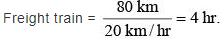So, Distance travelled = 4 × 60 or 6 × 40 = 240 km.

QUESTION: 8

A daily train is to be introduced between station A and station B starting from each at 6 AM and the journey is to be completed in 42 hours. What is the number of trains needed in order to maintain the Shuttle Service?



Solution: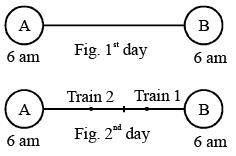Above given figures show that train 1 leaves station A on 1st day and train 2 leaves station B on 1st day. Both the trains don’t reach their destination even on the 2nd day after 24 hours as the journey completes in 42 hours.
∴ Two more trains need to be introduced at both the stations i.e., station A and B. So, 4 trains are needed in order to maintain the shuttle service.

QUESTION: 9

Four friends A, B, C and D need to cross a bridge. A maximum of two persons can cross it at a time. It is night and they just have one lamp. Persons that cross the bridge must carry the lamp to find the way. A pair must walk together at the speed of slower person. After crossing the bridge, the person having faster speed in the pair will return with the lamp each time to accompany another person in the group. Finally, the lamp has to be returned at the original place and the person who returns the lamp has to cross the bridge again without lamp. To cross the bridge, the time taken by them is as follows :
A : 1 minute, B : 2 minutes, C : 7 minutes and D : 10 minutes.
What is the total minimum time required by all the friends to cross the bridge?



Solution:

Here, A takes the shortest time to cross the bridge i.e. 1 min. And, D takes 10 min, C takes 7 min and B takes 2 min to cross the bridge.
So, 4 friends can cross the bridge in minimum time in the following ways.
1. A + B crossing and A returning : (2 + 1) min
2. A + C crossing and A returning : (7 + 1) min
3. A + D crossing and A returning : (10 + 1) min
4. A crossing the bridge for the last time : 1 min.
Hence, total minimum time = 3 + 8 + 11 + 1 = 23 min.

QUESTION: 10

A and B walk around a circular park. They start at 8 a.m. from the same point in the opposite directions. A and B walk at a speed of 2 rounds per hour and 3 rounds per hour respectively. How many times shall they cross each other after 8·00 a.m. and before 9.30. a.m.?



Solution:

Here, A and B are moving in opposite directions. So, Relative speed = 2 + 3 = 5 rounds/hour So, they cross each other 5 times in an hour and 2 times in half an hour.
Hence, they cross each other 7 times before 9 : 30 a.m.